x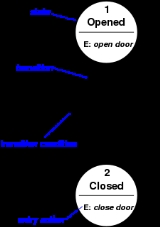Finite state machineEncyclopedia
A finite-state machine (FSM) or finite-state automaton (plural: automata), or simply a state machine
Abstract machine
An abstract machine, also called an abstract computer, is a theoretical model of a computer hardware or software system used in automata theory...

, is a mathematical model
Mathematical model
A mathematical model is a description of a system using mathematical concepts and language. The process of developing a mathematical model is termed mathematical modeling. Mathematical models are used not only in the natural sciences and engineering disciplines A mathematical model is a...

used to design computer programs and digital logic circuits. It is conceived as an abstract machine that can be in one of a finite number of states
State (computer science)
In computer science and automata theory, a state is a unique configuration of information in a program or machine. It is a concept that occasionally extends into some forms of systems programming such as lexers and parsers....

. The machine is in only one state at a time; the state it is in at any given time is called the current state. It can change from one state to another when initiated by a triggering event or condition, this is called a transition. A particular FSM is defined by a list of the possible states it can transition to from each state, and the triggering condition for each transition.

Finite-state machines can model a large number of problems, among which are electronic design automation
Electronic design automation
Electronic design automation is a category of software tools for designing electronic systems such as printed circuit boards and integrated circuits...

, communication protocol design, parsing
Parsing
In computer science and linguistics, parsing, or, more formally, syntactic analysis, is the process of analyzing a text, made of a sequence of tokens , to determine its grammatical structure with respect to a given formal grammar...

and other engineering applications. In biology
Biology
Biology is a natural science concerned with the study of life and living organisms, including their structure, function, growth, origin, evolution, distribution, and taxonomy. Biology is a vast subject containing many subdivisions, topics, and disciplines...

and artificial intelligence
Artificial intelligence
Artificial intelligence is the intelligence of machines and the branch of computer science that aims to create it. AI textbooks define the field as "the study and design of intelligent agents" where an intelligent agent is a system that perceives its environment and takes actions that maximize its...

research, state machines or hierarchies of state machines are sometimes used to describe neurological systems
Neurology
Neurology is a medical specialty dealing with disorders of the nervous system. Specifically, it deals with the diagnosis and treatment of all categories of disease involving the central, peripheral, and autonomic nervous systems, including their coverings, blood vessels, and all effector tissue,...

and in linguistics
Linguistics
Linguistics is the scientific study of human language. Linguistics can be broadly broken into three categories or subfields of study: language form, language meaning, and language in context....

—to describe the grammars of natural languages.

## Concepts and vocabulary

A state describes a behavioral node of the system in which it is waiting for a trigger to execute a transition. Typically a state is introduced when the system does not react the same way to the same trigger. In the example of a car radio system, when listening to the radio (in the radio state), the "next" stimulus means going to the next station. But when the system is in the CD state, the "next" stimulus means going to the next track. The same stimulus triggers different actions depending on the current state. In some Finite-state machine representations, it is also possible to associate actions to a state:
• Entry action: which is performed when entering the state,
• Exit action: which is performed when exiting the state.

A transition is a set of actions to be executed when a condition is fulfilled or when an event is received.

## Representations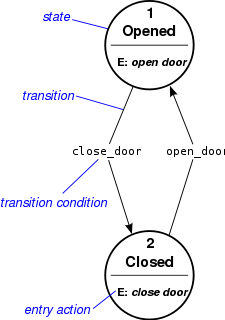For an introduction, see State diagram
State diagram
A state diagram is a type of diagram used in computer science and related fields to describe the behavior of systems. State diagrams require that the system described is composed of a finite number of states; sometimes, this is indeed the case, while at other times this is a reasonable abstraction...

.

### State/Event table

Several state transition table
State transition table
In automata theory and sequential logic, a state transition table is a table showing what state a finite semiautomaton or finite state machine will move to, based on the current state and other inputs...

types are used. The most common representation is shown below: the combination of current state (e.g. B) and input (e.g. Y) shows the next state (e.g. C). The complete actions information is not directly described in the table. Which can be added only using footnotes. An FSM definition including the full actions information is possible using state tables (see also VFSM).
 Current state → Input ↓ Input X Input Y State A State B State C ... ... ... ... State C ... ... ... ...

### UML state machines

The Unified Modeling Language
Unified Modeling Language
Unified Modeling Language is a standardized general-purpose modeling language in the field of object-oriented software engineering. The standard is managed, and was created, by the Object Management Group...

has a notation for describing state machines. UML state machine
UML state machine
UML state machine is a significantly enhanced realization of the mathematical concept of a finite automaton in Computer Science applications as expressed in the Unified Modeling Language notation....

s overcome the limitations of traditional finite state machines while retaining their main benefits. UML state machines introduce the new concepts of hierarchically nested states and orthogonal regions, while extending the notion of actions. UML state machines have the characteristics of both Mealy machine
Mealy machine
In the theory of computation, a Mealy machine is a finite-state machine whose output values are determined both by its current state and the current inputs. The outputs change asynchronously with respect to the clock, meaning that the outputs change at unpredictable times, making timing analysis...

s and Moore machine
Moore machine
In the theory of computation, a Moore machine is a finite-state machine, whose output values are determined solely by its current state.-Name:The Moore machine is named after Edward F...

s. They support actions that depend on both the state of the system and the triggering event, as in Mealy machines, as well as entry and exit actions, which are associated with states rather than transitions, as in Moore machines.

### SDL state machines

The Specification and Description Language
Specification and Description Language
Specification and Description Language is a specification language targeted at the unambiguous specification and description of the behaviour of reactive and distributed systems.- Overview :It is defined by the ITU-T...

is a standard from ITU and is one of the best languages to describe state machines because it includes graphical symbols to describe actions in the transition:
• send an event
• start a timer
• cancel a timer
• start another concurrent state machine
• decision

SDL embeds basic data types called Abstract Data Types, an action language, and an execution semantic in order to make the finite state machine executable.

### Other state diagrams

There are a large number of variants to represent an FSM such as the one in figure 3.

## Usage

In addition to their use in modeling reactive systems presented here, finite state automata are significant in many different areas, including electrical engineering
Electrical engineering
Electrical engineering is a field of engineering that generally deals with the study and application of electricity, electronics and electromagnetism. The field first became an identifiable occupation in the late nineteenth century after commercialization of the electric telegraph and electrical...

, linguistics
Linguistics
Linguistics is the scientific study of human language. Linguistics can be broadly broken into three categories or subfields of study: language form, language meaning, and language in context....

, computer science
Computer science
Computer science or computing science is the study of the theoretical foundations of information and computation and of practical techniques for their implementation and application in computer systems...

, philosophy
Philosophy
Philosophy is the study of general and fundamental problems, such as those connected with existence, knowledge, values, reason, mind, and language. Philosophy is distinguished from other ways of addressing such problems by its critical, generally systematic approach and its reliance on rational...

, biology
Biology
Biology is a natural science concerned with the study of life and living organisms, including their structure, function, growth, origin, evolution, distribution, and taxonomy. Biology is a vast subject containing many subdivisions, topics, and disciplines...

, mathematics, and logic
Logic
In philosophy, Logic is the formal systematic study of the principles of valid inference and correct reasoning. Logic is used in most intellectual activities, but is studied primarily in the disciplines of philosophy, mathematics, semantics, and computer science...

. Finite state machines are a class of automata studied in automata theory
Automata theory
In theoretical computer science, automata theory is the study of abstract machines and the computational problems that can be solved using these machines. These abstract machines are called automata...

and the theory of computation
Theory of computation
In theoretical computer science, the theory of computation is the branch that deals with whether and how efficiently problems can be solved on a model of computation, using an algorithm...

.
In computer science, finite state machines are widely used in modeling of application behavior, design of hardware digital systems, software engineering, compilers, network protocols, and the study of computation and languages.

## Classification

There are two different groups of state machines: Acceptors/Recognizers and Transducers.

### Acceptors and recognizers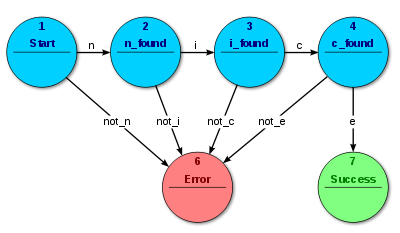Acceptors and recognizers (also sequence detectors) produce a binary output, saying either yes or no to answer whether the input is accepted by the machine or not. All states of the FSM are said to be either accepting or not accepting. At the time when all input is processed, if the current state is an accepting state, the input is accepted; otherwise it is rejected. As a rule the input are symbols (characters); actions are not used. The example in figure 4 shows a finite state machine which accepts the word "nice". In this FSM the only accepting state is number 7.

The machine can also be described as defining a language, which would contain every word accepted by the machine but none of the rejected ones; we say then that the language is accepted by the machine. By definition, the languages accepted by FSMs are the regular language
Regular language
In theoretical computer science and formal language theory, a regular language is a formal language that can be expressed using regular expression....

s—that is, a language is regular if there is some FSM that accepts it.

#### Start state

The start state is usually shown drawn with an arrow "pointing at it from any where" (Sipser (2006) p. 34).

#### Accept (or final) states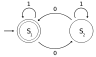Accept states (also referred to as accepting or final states) are those at which the machine reports that the input string, as processed so far, is a member of the language it accepts. It is usually represented by a double circle.

An example of an accepting state appears in the diagram to the right: a deterministic finite automaton (DFA) that detects whether the binary
Binary numeral system
The binary numeral system, or base-2 number system, represents numeric values using two symbols, 0 and 1. More specifically, the usual base-2 system is a positional notation with a radix of 2...

input string contains an even number of 0's.

S1 (which is also the start state) indicates the state at which an even number of 0's has been input. S1 is therefore an accepting state. This machine will finish in an accept state, if the binary string contains an even number of 0's (including any binary string containing no 0's). Examples of strings accepted by this DFA are epsilon (the empty string), 1, 11, 11..., 00, 010, 1010, 10110, etc...

### Transducers

Transducers
Finite state transducer
A finite state transducer is a finite state machine with two tapes: an input tape and an output tape. This contrasts with an ordinary finite state automaton , which has a single tape.-Overview:...

generate output based on a given input and/or a state using actions. They are used for control applications and in the field of computational linguistics
Computational linguistics
Computational linguistics is an interdisciplinary field dealing with the statistical or rule-based modeling of natural language from a computational perspective....

. Here two types are distinguished:

Moore machine
Moore machine
In the theory of computation, a Moore machine is a finite-state machine, whose output values are determined solely by its current state.-Name:The Moore machine is named after Edward F...

: The FSM uses only entry actions, i.e., output depends only on the state. The advantage of the Moore model is a simplification of the behaviour. Consider an elevator door. The state machine recognizes two commands: "command_open" and "command_close" which trigger state changes. The entry action (E:) in state "Opening" starts a motor opening the door, the entry action in state "Closing" starts a motor in the other direction closing the door. States "Opened" and "Closed" stop the motor when fully opened or closed. They signal to the outside world (e.g., to other state machines) the situation: "door is open" or "door is closed".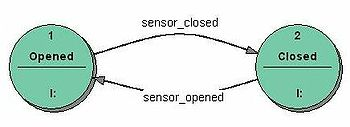Mealy machine
Mealy machine
In the theory of computation, a Mealy machine is a finite-state machine whose output values are determined both by its current state and the current inputs. The outputs change asynchronously with respect to the clock, meaning that the outputs change at unpredictable times, making timing analysis...

: The FSM uses only input actions, i.e., output depends on input and state. The use of a Mealy FSM leads often to a reduction of the number of states. The example in figure 7 shows a Mealy FSM implementing the same behaviour as in the Moore example (the behaviour depends on the implemented FSM execution model and will work, e.g., for virtual FSM
Virtual finite state machine
A virtual finite state machine is a finite state machine defined in a virtual environment. The VFSM concept provides a software specification method to describe the behaviour of a control system using assigned names of input control properties and of output actions.The VFSM method introduces an...

but not for event driven FSM). There are two input actions (I:): "start motor to close the door if command_close arrives" and "start motor in the other direction to open the door if command_open arrives". The "opening" and "closing" intermediate states are not shown.

In practice[of what?] mixed models are often used.

More details about the differences and usage of Moore and Mealy models, including an executable example, can be found in the external technical note "Moore or Mealy model?"

### Determinism

A further distinction is between deterministic (DFA
Deterministic finite state machine
In the theory of computation and automata theory, a deterministic finite state machine—also known as deterministic finite automaton —is a finite state machine accepting finite strings of symbols. For each state, there is a transition arrow leading out to a next state for each symbol...

) and non-deterministic (NFA
Nondeterministic finite state machine
In the automata theory, a nondeterministic finite state machine or nondeterministic finite automaton is a finite state machine where from each state and a given input symbol the automaton may jump into several possible next states...

, GNFA) automata. In deterministic automata, every state has exactly one transition for each possible input. In non-deterministic automata, an input can lead to one, more than one or no transition for a given state. This distinction is relevant in practice, but not in theory, as there exists an algorithm (the powerset construction
Powerset construction
In the theory of computation and Automata theory, the powerset construction or subset construction is a standard method for converting a nondeterministic finite automaton into a deterministic finite automaton which recognizes the same formal language...

) which can transform any NFA into a more complex DFA with identical functionality.

The FSM with only one state is called a combinatorial FSM and uses only input actions. This concept is useful in cases where a number of FSM are required to work together, and where it is convenient to consider a purely combinatorial part as a form of FSM to suit the design tools.

## Alternative semantics

There are other sets of semantics available to represent state machines. For example, there are tools for modeling and designing logic for embedded controllers. They combine hierarchical state machines, flow graphs, and truth tables into one language, resulting in a different formalism and set of semantics. Figure 8 illustrates this mix of state machines and flow graphs with a set of states to represent the state of a stopwatch and a flow graph to control the ticks of the watch. These charts, like Harel's original state machines, support hierarchically nested states, orthogonal regions, state actions, and transition actions.

## FSM logic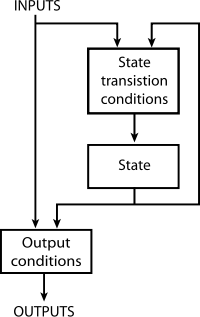The next state and output of an FSM is a function of the input and of the current state. The FSM logic is shown in Figure 8.

## Mathematical model

In accordance with the general classification, the following formal definitions are found:
• A deterministic finite state machine or acceptor deterministic finite state machine is a quintuple
Tuple
In mathematics and computer science, a tuple is an ordered list of elements. In set theory, an n-tuple is a sequence of n elements, where n is a positive integer. There is also one 0-tuple, an empty sequence. An n-tuple is defined inductively using the construction of an ordered pair...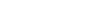, where:
•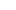is the input alphabet (a finite, non-empty set of symbols).
•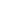is a finite, non-empty set of states.
•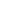is an initial state, an element of.
•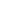is the state-transition function: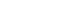(in a nondeterministic finite state machine
Nondeterministic finite state machine
In the automata theory, a nondeterministic finite state machine or nondeterministic finite automaton is a finite state machine where from each state and a given input symbol the automaton may jump into several possible next states...

it would be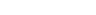, i.e.,would return a set of states).
•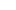is the set of final states, a (possibly empty) subset of.

For both deterministic and non-deterministic FSMs, it is conventional to allowto be a partial function
Partial function
In mathematics, a partial function from X to Y is a function ƒ: X' → Y, where X' is a subset of X. It generalizes the concept of a function by not forcing f to map every element of X to an element of Y . If X' = X, then ƒ is called a total function and is equivalent to a function...

, i.e.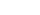does not have to be defined for every combination ofand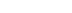. If an FSM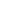is in a state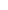, the next symbol isandis not defined, thencan announce an error (i.e. reject the input). This is useful in definitions of general state machines, but less useful when transforming the machine. Some algorithms in their default form may require total functions.

A finite-state machine is a restricted Turing machine
Turing machine
A Turing machine is a theoretical device that manipulates symbols on a strip of tape according to a table of rules. Despite its simplicity, a Turing machine can be adapted to simulate the logic of any computer algorithm, and is particularly useful in explaining the functions of a CPU inside a...

where the head can only perform "read" operations, and always moves from left to right.
• A finite state transducer
Finite state transducer
A finite state transducer is a finite state machine with two tapes: an input tape and an output tape. This contrasts with an ordinary finite state automaton , which has a single tape.-Overview:...

is a sextuple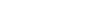, where:
•is the input alphabet (a finite non empty set of symbols).
•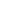is the output alphabet (a finite, non-empty set of symbols).
•is a finite, non-empty set of states.
•is the initial state, an element of. In a nondeterministic finite state machine
Nondeterministic finite state machine
In the automata theory, a nondeterministic finite state machine or nondeterministic finite automaton is a finite state machine where from each state and a given input symbol the automaton may jump into several possible next states...

,is a set of initial states.
•is the state-transition function:.
•is the output function.

If the output function is a function of a state and input alphabet (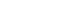) that definition corresponds to the Mealy model, and can be modelled as a Mealy machine
Mealy machine
In the theory of computation, a Mealy machine is a finite-state machine whose output values are determined both by its current state and the current inputs. The outputs change asynchronously with respect to the clock, meaning that the outputs change at unpredictable times, making timing analysis...

. If the output function depends only on a state (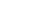) that definition corresponds to the Moore model, and can be modelled as a Moore machine
Moore machine
In the theory of computation, a Moore machine is a finite-state machine, whose output values are determined solely by its current state.-Name:The Moore machine is named after Edward F...

. A finite-state machine with no output function at all is known as a semiautomaton
Semiautomaton
In mathematics and theoretical computer science, a semiautomaton is an automaton having only an input, and no output. It consists of a set Q of states, a set Σ called the input alphabet, and a function T: Q × Σ → Q called the transition function.Associated to any semiautomaton is a monoid called...

or transition system.

If we disregard the first output symbol of a Moore machine,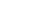, then it can be readily converted to an output-equivalent Mealy machine by setting the output function of every Mealy transition (i.e. labeling every edge) with the output symbol given of the destination Moore state. The converse transformation is less straightforward because a Mealy machine state may have different output labels on its incoming transitions (edges). Every such state needs to be split in multiple Moore machine states, one for every incident output symbol.

## Optimization

Optimizing an FSM means finding the machine with the minimum number of states that performs the same function. The fastest known algorithm doing this is the Hopcroft minimization algorithm. Other techniques include using an implication table
Implication table
An implication table is a tool used to facilitate the minimization of states in a state machine. The concept is to start assuming that every state may be able to combine with every other state, then eliminate combinations that are not possible...

, or the Moore reduction procedure
Moore reduction procedure
In computer science, the Moore reduction procedure is a method used for DFA minimization.The concept is to start assuming that every state may be able to combine with every other state, then separate distinguishable states into separate groups called equivalence partitions...

. Additionally, acyclic FSAs can be optimized using a simple bottom up algorithm.

### Hardware applications

In a digital circuit
Digital circuit
Digital electronics represent signals by discrete bands of analog levels, rather than by a continuous range. All levels within a band represent the same signal state...

, an FSM may be built using a programmable logic device
Programmable logic device
A programmable logic device or PLD is an electronic component used to build reconfigurable digital circuits. Unlike a logic gate, which has a fixed function, a PLD has an undefined function at the time of manufacture...

, a programmable logic controller
Programmable logic controller
A programmable logic controller or programmable controller is a digital computer used for automation of electromechanical processes, such as control of machinery on factory assembly lines, amusement rides, or light fixtures. PLCs are used in many industries and machines...

, logic gate
Logic gate
A logic gate is an idealized or physical device implementing a Boolean function, that is, it performs a logical operation on one or more logic inputs and produces a single logic output. Depending on the context, the term may refer to an ideal logic gate, one that has for instance zero rise time and...

s and flip flops
Flip-flop (electronics)
In electronics, a flip-flop or latch is a circuit that has two stable states and can be used to store state information. The circuit can be made to change state by signals applied to one or more control inputs and will have one or two outputs. It is the basic storage element in sequential logic...

or relay
Relay
A relay is an electrically operated switch. Many relays use an electromagnet to operate a switching mechanism mechanically, but other operating principles are also used. Relays are used where it is necessary to control a circuit by a low-power signal , or where several circuits must be controlled...

s. More specifically, a hardware implementation requires a register
Processor register
In computer architecture, a processor register is a small amount of storage available as part of a CPU or other digital processor. Such registers are addressed by mechanisms other than main memory and can be accessed more quickly...

to store state variables, a block of combinational logic which determines the state transition, and a second block of combinational logic that determines the output of an FSM. One of the classic hardware implementations is the Richards controller.

Mealy and Moore machines produce logic with asynchronous output, because there is a propagation delay between the flip-flop and output. This causes slower operating frequencies in FSM. A Mealy or Moore machine can be convertible to a FSM which output is directly from a flip-flop, which makes the FSM run at higher frequencies. This kind of FSM is sometimes called Medvedev FSM. A counter is the simplest form of this kind of FSM.

### Software applications

The following concepts are commonly used to build software applications with finite state machines:
• Automata-based programming
Automata-Based Programming
Automata-based programming is a programming paradigm in which the program or its part is thought of as a model of a finite state machine or any other formal automaton...

• Event driven FSM
• Virtual FSM (VFSM)
Virtual finite state machine
A virtual finite state machine is a finite state machine defined in a virtual environment. The VFSM concept provides a software specification method to describe the behaviour of a control system using assigned names of input control properties and of output actions.The VFSM method introduces an...

• Abstract state machines
Abstract State Machines
In computer science, an abstract state machine is a state machine operating on states which are arbitrary data structures .The ASM Method is a practical and scientifically well-founded systems engineering method which bridges...

(ASM)
• Artificial intelligence
Artificial intelligence
Artificial intelligence is the intelligence of machines and the branch of computer science that aims to create it. AI textbooks define the field as "the study and design of intelligent agents" where an intelligent agent is a system that perceives its environment and takes actions that maximize its...

(AI)
• Abstract State Machine Language
Abstract State Machine Language
Abstract State Machine Language is a programming language based on the Abstract State Machines formal method and developed by Microsoft. AsmL is a functional language ....

(AsmL)
• Control system
Control system
A control system is a device, or set of devices to manage, command, direct or regulate the behavior of other devices or system.There are two common classes of control systems, with many variations and combinations: logic or sequential controls, and feedback or linear controls...

• Control table
Control table
Control tables are tables that control the program flow or play a major part in program control. There are no rigid rules concerning the structure or content of a control table - its only qualifying attribute is its ability to direct program flow in some way through its 'execution' by an associated...

• Decision table
Decision table
Decision tables are a precise yet compact way to model complicated logic.Decision tables, like flowcharts and if-then-else and switch-case statements, associate conditions with actions to perform, but in many cases do so in a more elegant way....

s
• DEVS
DEVS
DEVS abbreviating Discrete Event System Specification is a modular and hierarchical formalism for modeling and analyzing general systems that can be discrete event systems which might be described by state transition tables, and continuous state systems which might be described by differential...

: Discrete Event System Specification
• Extended finite-state machine (EFSM)
• Finite state machine with datapath
FSMD
FSMD is called as Finite State Machine with Datapath. It is mathematical abstraction sometimes used to design digital logic or computer programs.An FSMD is a digital system composed of a finite-state machine, which controls the program flow, and a `datapath`, which performs operations.FSMD is more...

• Hidden Markov model
Hidden Markov model
A hidden Markov model is a statistical Markov model in which the system being modeled is assumed to be a Markov process with unobserved states. An HMM can be considered as the simplest dynamic Bayesian network. The mathematics behind the HMM was developed by L. E...

• Petri net
Petri net
A Petri net is one of several mathematical modeling languages for the description of distributed systems. A Petri net is a directed bipartite graph, in which the nodes represent transitions and places...

• Pushdown automaton
Pushdown automaton
In automata theory, a pushdown automaton is a finite automaton that can make use of a stack containing data.- Operation :Pushdown automata differ from finite state machines in two ways:...

• Quantum finite automata
Quantum finite automata
In quantum computing, quantum finite automata or QFA are a quantum analog of probabilistic automata. They are related to quantum computers in a similar fashion as finite automata are related to Turing machines. Several types of automata may be defined, including measure-once and measure-many automata...

(QFA)
• Recognizable language
Recognizable language
In mathematics and computer science, a recognizable language is a formal language that is recognized by a finite state machine. Equivalently, a recognizable language is one for which the family of quotients for the syntactic relation is finite.-Definition:...

• Sequential logic
Sequential logic
In digital circuit theory, sequential logic is a type of logic circuit whose output depends not only on the present input but also on the history of the input. This is in contrast to combinational logic, whose output is a function of, and only of, the present input...

• Specification and Description Language
Specification and Description Language
Specification and Description Language is a specification language targeted at the unambiguous specification and description of the behaviour of reactive and distributed systems.- Overview :It is defined by the ITU-T...

• State diagram
State diagram
A state diagram is a type of diagram used in computer science and related fields to describe the behavior of systems. State diagrams require that the system described is composed of a finite number of states; sometimes, this is indeed the case, while at other times this is a reasonable abstraction...

• Transition system
• Tree automaton
Tree automaton
A tree automaton is a type of state machine. Tree automata deal with tree structures, rather than the strings of more conventional state machines.The following article deals with branching tree automata, which correspond to regular languages of trees...

• Turing machine
Turing machine
A Turing machine is a theoretical device that manipulates symbols on a strip of tape according to a table of rules. Despite its simplicity, a Turing machine can be adapted to simulate the logic of any computer algorithm, and is particularly useful in explaining the functions of a CPU inside a...

• UML state machine
UML state machine
UML state machine is a significantly enhanced realization of the mathematical concept of a finite automaton in Computer Science applications as expressed in the Unified Modeling Language notation....

• SCXML
SCXML
SCXML stands for State Chart XML: State Machine Notation for Control Abstraction. It is an XML-based markup language which provides a generic state-machine based execution environment based on Harel statecharts.SCXML is able to describe complex state-machines...

• OpenGL
OpenGL
OpenGL is a standard specification defining a cross-language, cross-platform API for writing applications that produce 2D and 3D computer graphics. The interface consists of over 250 different function calls which can be used to draw complex three-dimensional scenes from simple primitives. OpenGL...

### General

• Wagner, F., "Modeling Software with Finite State Machines: A Practical Approach", Auerbach Publications, 2006, ISBN 0-8493-8086-3.
• ITU-T, Recommendation Z.100 Specification and Description Language (SDL)
• Samek, M., Practical Statecharts in C/C++, CMP Books, 2002, ISBN 1-57820-110-1.
• Samek, M., Practical UML Statecharts in C/C++, 2nd Edition, Newnes, 2008, ISBN 0-7506-8706-1.
• Gardner, T., Advanced State Management, 2007
• Cassandras, C., Lafortune, S., "Introduction to Discrete Event Systems". Kluwer, 1999, ISBN 0-7923-8609-4.
• Timothy Kam, Synthesis of Finite State Machines: Functional Optimization. Kluwer Academic Publishers, Boston 1997, ISBN 0-7923-9842-4
• Tiziano Villa, Synthesis of Finite State Machines: Logic Optimization. Kluwer Academic Publishers, Boston 1997, ISBN 0-7923-9892-0
• Carroll, J., Long, D., Theory of Finite Automata with an Introduction to Formal Languages. Prentice Hall, Englewood Cliffs, 1989.
• Kohavi, Z., Switching and Finite Automata Theory. McGraw-Hill, 1978.
• Gill, A., Introduction to the Theory of Finite-state Machines. McGraw-Hill, 1962.
• Ginsburg, S., An Introduction to Mathematical Machine Theory. Addison-Wesley, 1962.

### Finite state machines (automata theory) in theoretical computer science

Extensive, wide-ranging book meant for specialists, written for both theoretical computer scientists as well as electrical engineers. With detailed explanations of state minimization techniques, FSMs, Turing machines, Markov processes, and undecidability. Excellent treatment of Markov processes. Excellent. Has been in print in various editions and reprints since 1974 (1974, 1980, 1989, 1999). Approaches Church-Turing thesis from three angles: levels of finite automata as acceptors of formal languages, primitive and partial recursive theory, and power of bare-bones programming languages to implement algorithms, all in one slim volume. An excellent book centered around the issues of machine-interpretation of "languages", NP-Completeness, etc. Distinctly different and less intimidating than the first edition. Minsky spends pages 11–20 defining what a "state" is in context of FSMs. His state diagram convention is unconventional. Excellent, i.e., relatively readable, sometimes funny. Abstract algebra is at the core of the book, rendering it advanced and less accessible than other texts. cf Finite state machines (finite automata) in chapter 29.

### Abstract state machines in theoretical computer science

• Yuri Gurevich (2000), Sequential Abstract State Machines Capture Sequential Algorithms, ACM Transactions on Computational Logic, vl. 1, no. 1 (July 2000), pages 77–111. http://research.microsoft.com/~gurevich/Opera/141.pdf

### Machine learning using finite-state algorithms

A broad brush but quite thorough and sometimes difficult, meant for computer scientists and engineers. Chapter 13 Reinforcement Learning deals with robot-learning involving state-machine-like algorithms.

### Hardware engineering: state minimization and synthesis of sequential circuits

Extensive, wide-ranging book meant for specialists, written for both theoretical computer scientists as well as electrical engineers. With detailed explanations of state minimization techniques, FSMs, Turing machines, Markov processes, and undecidability. Excellent treatment of Markov processes. Meant for electrical engineers. More focused, less demanding than his earlier book. His treatment of computers is out-dated. Interesting take on definition of "algorithm". Meant for hardware electrical engineers. With detailed explanations of state minimization techniques and synthesis techniques for design of combinatory logic circuits. Meant for hardware electrical engineers. Excellent explanations of state minimization techniques and synthesis techniques for design of combinatory and sequential logic circuits.

### Finite Markov chain processes

"We may think of a Markov chain
Markov chain
A Markov chain, named after Andrey Markov, is a mathematical system that undergoes transitions from one state to another, between a finite or countable number of possible states. It is a random process characterized as memoryless: the next state depends only on the current state and not on the...

as a process that moves successively through a set of states s1, s2, ..., sr. ... if it is in state si it moves on to the next stop to state sj with probability pij. These probabilities can be exhibited in the form of a transition matrix" (Kemeny (1959), p. 384)

Finite Markov-chain processes are also known as subshifts of finite type.
Extensive, wide-ranging book meant for specialists, written for both theoretical computer scientists as well as electrical engineers. With detailed explanations of state minimization techniques, FSMs, Turing machines, Markov processes, and undecidability. Excellent treatment of Markov processes. Classical text. cf. Chapter 6 "Finite Markov Chains".